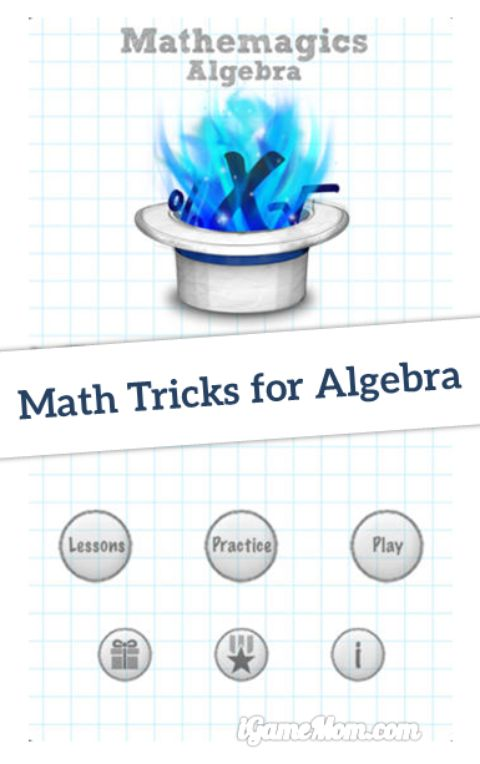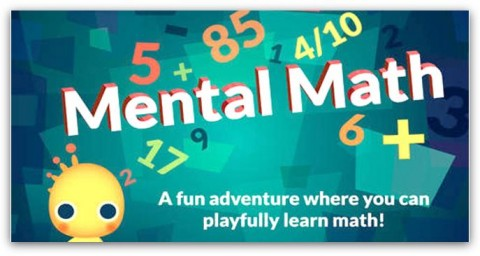≡ Categories

# Learn Math Tricks for Algebra with MathemagicsLearning math is fun. It should be. Today we share an app that teaches kids math tricks for algebra: Mathemagics. It is the 2nd app in the series. The first Mathemagics app focuses on math tricks on mental math calculations, like adding and multiplying two digit numbers, or dividing a number by 7. This 2nd app in the series teaches math tricks on algebra. It is a great way to learn thinking differently and encourage kids to find better solutions when solving problems.As I look through the solutions included the app, I see that it really is teaching kids different approaches in solving algebraic problems, or looking at the same problem from a different angle. To goal is to accelerate algebraic calculation and accuracy as kids expand their knowledge with the techniques, which are usually different from the approaches taught in the text books used in schools.

Even kids won’t solve the algebra problems faster with these techniques, it is still good to know there are different ways to solve those problems. Based on my experience, once you can see a problems from a different angle, you start having better understanding of the problem. When kids have a real understand of the issue, even though they don’t use the techniques taught to them, they will be able to solve the problems faster and more accurately, using the approach they feel comfortable.

The techniques covered in the app include:

* Calculate 10% of Any Number
* Calculate 9% of Any Number
* Calculate 11% of Any Number
* Percentages of Numbers Less Than 100
* Percentages of Numbers From 100-999
* Multiply Two Polynomials
* Multiply Two Trinomials
* Multiply Large Polynomials
* Check Polynomial Multiplication
* Basic Algebraic Division
* Intermediate Algebraic Division
* Verify Algebraic Division
* Solve Simultaneous Equations
* Special Case Simultaneous Equations

Each technique lesson has clear step descriptions with animated examples that can be watched as often as needed in order to ensure that kids grasp the concept.

IF you have a child who is learning algebra, this is a good app to have to expand or deepen kids’ understanding of algebra problems.

Mathemagics for Algebra is available on iPhone, iPod and iPad. It is \$2.99 on App Store.

If you would like to check it out or purchase it, please use the App Store link provided below. The cost is the same to you, but iGameMom gets a small percentage. Thanks for your support! Note: The link works for all countries.Looking for math tricks for younger kids? Try this Mental Math App. It teaches kids the skills they need for addition and multiplication problems.{ 1 comment… add one }